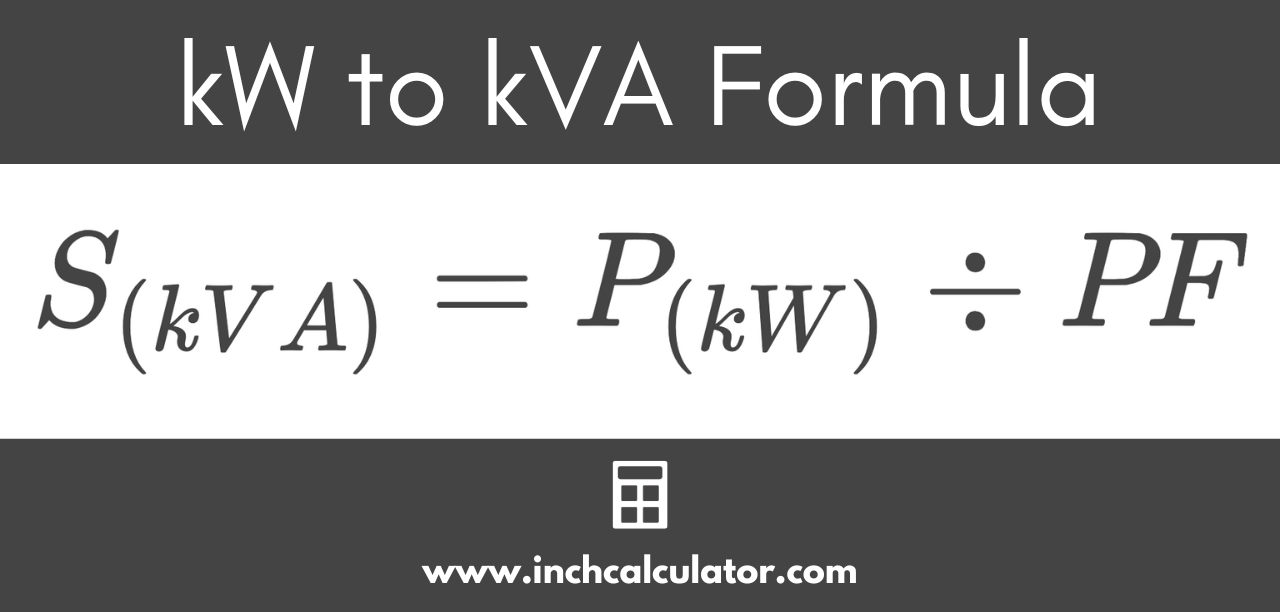# Kilowatts (kW) to Kilovolt-Amps (kVA) Conversion Calculator

Convert kilowatts (kW) to kilovolt-amps (kVA) by entering the power and power factor in the form below.

kW

## Kilovolt-Amps Results:

kVA
Learn how we calculated this below

## How to Convert KW to kVA

Kilowatts and kilovolt-amps are both a measure of power, but they’re a little different. Kilowatts (kW) measure the real power in an electrical circuit, while kilovolt-amps (kVA) measure the apparent power.

In electrical equipment with an inductive characteristic, such as a generator or transformer, the real power and apparent power are often not equal.

Real power is the amount of power that does real work in the circuit and is always the same or less than the apparent power. Apparent power is determined by the magnitude of voltage and current that must be transmitted to the device.

For reactive (inductive and/or capacitive) loads, electrical energy is stored in the device and pushed back to the power supply as opposed to doing real work. This phenomenon is called reactive power and is measured in kilovolt-amps-reactive (kVAR). Therefore, typically we try to maximize the real power and minimize reactive power.

The ratio of real power to apparent power is called the power factor (PF) and is between 0 and 1, where 1 is a pure resistive load and both inductive and capacitive loads have a PF less than 1.

To convert from kilowatts to kilovolt-amps considering all of this, you’ll need a simple formula.

### kW to kVA Formula

The formula to convert kW to kVA accounting for the power factor is:

S(kVA) = P(kW) ÷ PF

The apparent power S in kVA is equal to the real power P in kW divided by the power factor PF.

To convert to kVA, substitute the power in kW and the equipment power factor in the formula above.For example, let’s find the apparent power in kVA for a 10 kW electric motor with a power factor of 0.8.

S(kVA) = 10 kW ÷ 0.8
S(kVA) = 12.5 kVA

In this example, 10 kilowatts with a power factor of 0.8 is equivalent to 12.5 kilovolt-amps.

## kW to kVA Conversion Chart

This chart shows kilovolt-amps (kVA) converted to kilowatt (kW) ratings for equipment with a power factor of 0.8.

Equivalent kW and kVA ratings given a power factor of 0.8.
kW kVA
5 kW 6.3 kVA
7.5 kW 9.4 kVA
10 kW 12.5 kVA
15 kW 18.7 kVA
20 kW 25 kVA
25 kW 31.3 kVA
30 kW 37.5 kVA
40 kW 50 kVA
50 kW 62.5 kVA
60 kW 75 kVA
75 kW 93.8 kVA
80 kW 100 kVA
100 kW 125 kVA
125 kW 156 kVA
150 kW 187 kVA
175 kW 219 kVA
200 kW 250 kVA
250 kW 312 kVA
300 kW 375 kVA
350 kW 438 kVA
400 kW 500 kVA
500 kW 625 kVA
600 kW 750 kVA
700 kW 875 kVA
800 kW 1,000 kVA
900 kW 1,125 kVA
1,000 kW 1,250 kVA
1,250 kW 1,563 kVA
1,500 kW 1,875 kVA
1,750 kW 2,188 kVA
2,000 kW 2,500 kVA
2,250 kW 2,812 kVA

You might also be interested in our kVA to kW calculator.